# Texas Go Math Grade 5 Lesson 16.6 Answer Key Analyze Scatter Plots

Refer to our Texas Go Math Grade 5 Answer Key Pdf to score good marks in the exams. Test yourself by practicing the problems from Texas Go Math Grade 5 Lesson 16.6 Answer Key Analyze Scatter Plots.

## Texas Go Math Grade 5 Lesson 16.6 Answer Key Analyze Scatter Plots

Unlock the Problem

You can use a scatter plot to analyze the relationship between two sets of data.

Example 1

Students conducted an experiment about the relationship between the wingspans of paper airplanes and the distances they fly. How far did the paper airplane with a wingspan of 3 inches fly?
The point with x-coordinate 3 has y-coordinate ___.
So, the paper airplane with a wingspan of 3 inches
flew ___ feet.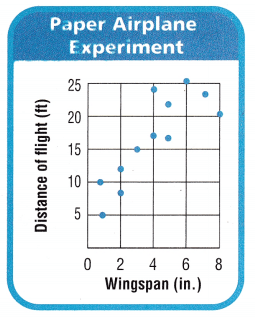15 feet.

Explanation:
Here, the point with x-coordinate 3 has y-coordinate 15.
So, the paper airplane with a wingspan of 3 inches flew 15 feet.

You can use scatter plots to make generalizations about data.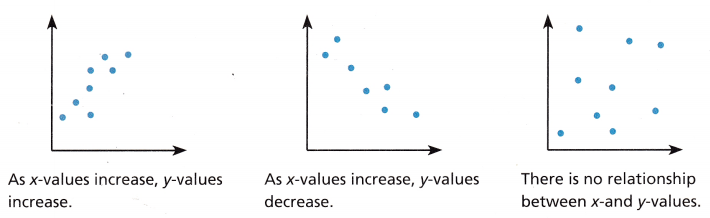• What happens to the distance the paper airplanes fly as the wingspan increases?

Math Talk
Mathematical Processes
Explain what the points (2, 9) and (2, 12) represent on the scatter plot.
When data shows a trend, you can make a prediction.

Example 2.
Solve a multi-step problem.
The scatter plot shows the relationship between monthly rainfall and sunglasses sales at a beach shop. About how many sunglasses do you think the shop will sell in a month with 5 inches of rain?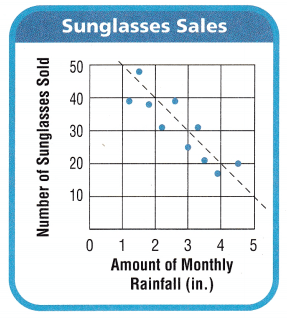• Draw a line to show the trend. Extend the line past x-coordinate 5.
• Use the line to estimate the coordinates of points that represent the number of sunglasses the shop will sell in a month with 5 inches of rain.
(5, ___)
So, the shop will sell about ___ sunglasses in a month with 5 inches of rain.

Share and Show

The scatter plot shows the relationship between the number of hours baseball players practiced and the number of hits they got. Use the scatter plot for 1-3.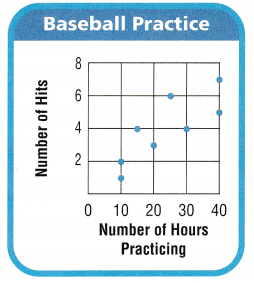Question 1.
How many hours did the player who got 6 hits practice?
• Write the ordered pair for the player who got 6 hits. (____, 6)
The player who got 6 hits practiced for ______ hours.

The player who got 6 hits practiced for 6 hours.

Explanation:
The number of hours did the player who got 6 hits practice is 25 hours.
The ordered pair for the player who got 6 hits is (25,6).

Question 2.
How many hits did the baseball players who practiced more than 30 hours get altogether?
20 hits.

Explanation:
The number of hits did the baseball players who practiced for more than 30 hours get all together is 1+2+3+4+6+4 which is 20 hits.

Question 3.
As the number of hours of baseball practice increases, does the number of hits increase, decrease, or stay about the same?
The number of hits is increasing.

Explanation:
As the number of hours of baseball practice increases the number of hits are increasing.

Question 4.
Generalize Suppose a scatter plot shows the relationship between the number of books in a library and the number of students who play soccer. As the number of books increases, does the number of students who play soccer increase? Does it decrease? Or is there no relationship between the two sets of data?
There is no relationship between the two sets of data as there is no relation between the number of books in a library and the number of students playing soccer. So there is no relationship between the two sets of data.

Question 5.
H.O.T. Sense or Nonsense Terry made a scatter plot to show the relationship between the number of weeks since a seed was planted and the height of the plant. Does this scatter plot make sense for the data? Explain.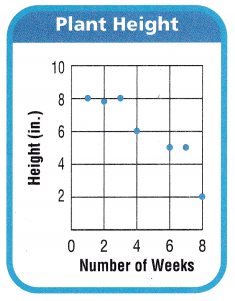Question 6.
Display Pam is making this scatter plot to show the relationship between the number of minutes students walk in a walkathon and the distance they walk. Graph 8 points on the scatter plot to show the relationship.Problem Solving

The scatter plot below shows the relationship between the number of camp counselors and the number of students in each group at camp. Use the scatter plot for 7—8.

Question 7.
Explain how the number of camp counselors is related to the number of students in each group at camp.

Question 8.
Multi-Step About what would you expect the number of students in each group at camp to be if there are 10 camp counselors? Explain.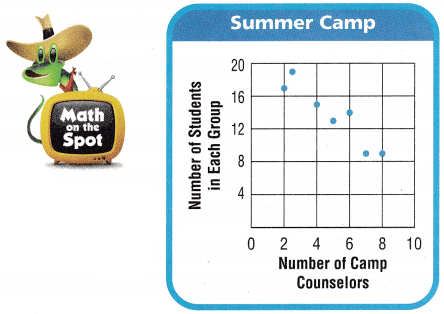Use the scatter plot for 9-10.

Question 9.
Molly collected data about the number of hours students studied and their scores on a test. The results are shown
in the scatter plot. Which statement about the data is true?
(A) As study hours increase, test scores decrease.
(B) As study hours increase, test scores increase.
(C) As study hours increase, test scores stay the same.
(D) As study hours decrease, test scores increase.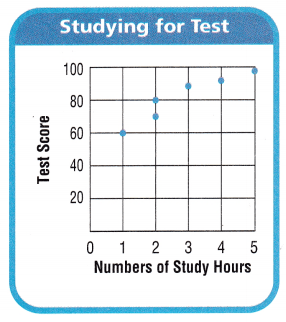B.

Explanation:
As study hours increase, test scores increase. This statement is true.

Question 10.
Which is a reasonable prediction of a test score for a student who studied for $$\frac{1}{2}$$ hour?
(A) 80
(B) 100
(C) 50
(D) 5
C.

Explanation:
The reasonable prediction of a test score for a student who studied for $$\frac{1}{2}$$ hour is 50 students.

Question 11.
Multi-Step Which relationship could be represented by this scatter plot?
(A) As the number of muffins in a box increases, the price increases.
(B) As the speed of a car increases, the distance the car travels increases.
(C) As the population of a city increases, the number of schools in the city increases.
(D) As the number of daylight hours increases, the number of night hours decreases.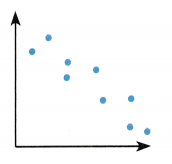D.

Explanation:
The relationship could be, As the number of daylight hours increases, the number of night hours decreases.

Texas Test Prep

Question 12.
As the area of a garden increases, what happens to the number of plants in a garden?
(A) The number of plants decreases.
(B) The number of plants stays the same.
(C) The number of plants increases faster.
(D) The number of plants increases.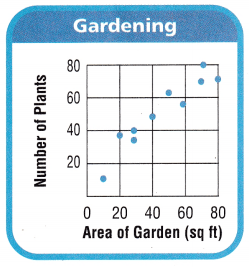D.

Explanation:
The area of a garden increases, the number of plants increases.

### Texas Go Math Grade 5 Lesson 16.6 Homework and Practice Answer Key

The scatter plot shows the relationship between the ages of the trees in Jane’s yard and the heights of the trees.
Use the scatter plot for 1-5.

Question 1.
What is the age of the tree that is 8 feet tall? ____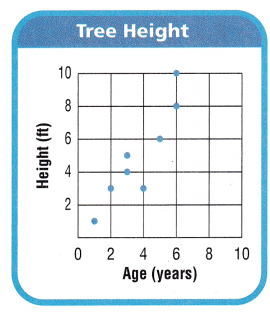The age is 6 years.

Explanation:
The age of the tree that is 8 feet tall is 6 years.

Question 2.
How many trees are 3 years old? ____
2 trees.

Explanation:
The number of trees that are 3 years old is 2 trees.

Question 3.
How many trees are more than 6 feet tall? _____
2 trees.

Explanation:
The number of trees that are more than 6 feet tall is 2 trees.

Question 4.
If a squirrel climbs to the top of each tree that is 6 years old, how far has the squirrel climbed? ____
8 ft and 10 ft.

Explanation:
If a squirrel climbs to the top of each tree that is 6 years old, so how far has the squirrel climbed is 8 ft and 10 ft.

Question 5.
Which height has the greatest frequency? ____

Problem Solving

Mr. Sanders has a box of candles. He makes a scatter plot to show the relationship between the number of minutes he burns each candle and the height of the candle when he blows It out. Use the scatter plot for 6-7.

Question 6.
Explain how the number of minutes the candle burns is related to the height of the candle.Question 7.
About what would you expect the height of a candle to be after burning for 2 hours 40 minutes? Explain.
The expected height will be about 4 cm.

Explanation:
The expected height of a candle to be after burning for 2 hours 40 minutes will be about 4 cm.

Texas Test Prep

Lesson Check

Question 8.
JoBeth draws a scatter plot that shows as the outside temperature increases, sales at her lemonade stand increase. Which best describes the scatter plot?
(A) The points show that as x-values increase, y-values increase.
(B) The points show that as x-values increase, y-values decrease.
(C) The points show that as x-values decrease, y-values stay the same.
(D) The points show that as x-values decrease, y-values increase.
A.

Explanation:
The best describes the scatter plot is the points show that as x-values increase, y-values increase.

Question 9.
Which of the following best describes a scatter plot that shows no relationship between the x-values and they-values?
(A) As x-values increase, the points are higher on the graph.
(B) As x-values decrease, the points are higher on the graph.
(C) The points are scattered around the graph.
(D) The points form a horizontal line.
B.

Explanation:
No relationship between the x-values and they-values is As x-values decrease, the points are higher on the graph.

The scatter plot shows the relationship between the hours people shopped online and the number of stores they visited. Use the scatter plot for 10—11.

Question 10.
Multi-Step Which statement best describes the relationship between time shopping online and the number of stores visited?
(A) As the number of hours increases, the number of stores decreases.
(B) As the number of hours increases, the number of stores increases.
(C) The number of hours and the number of stores are about the same.
(D) As the number of hours decreases, the number of stores increases.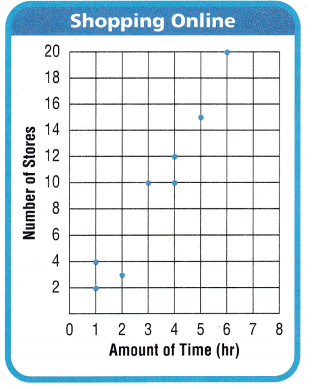B.

Explanation:
Here, the number of hours increases, the number of stores increases.

Question 11.
How much time did the person who visited 3 stores spend shopping online?
(A) 2hours
(B) 10 hours
(C) 4 hours
(D) 3 hours
B.

Explanation:
The number of times did the person who visited 3 stores spend shopping online is 10 hours.

Question 12.
Multi-Step Mr. Reynosa plans to shop online for 4 hours for two days in a row. What is a reasonable prediction of how many stores he will visit?
(A) 10
(B) 8
(C) 12
(D) 22